# Finding paths of length n in a graph

Suppose you have a non-directed graph, represented through its adjacency matrix. How would you discover how many paths of length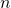link any two nodes?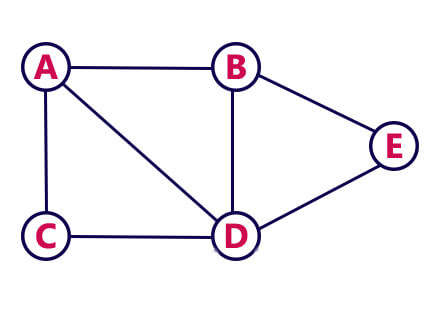For example, in the graph aside there is one path of length 2 that links nodes A and B (A-D-B). How can this be discovered from its adjacency matrix?

It turns out there is a beautiful mathematical way of obtaining this information! Although this is not the way it is used in practice, it is still very nice. In fact, Breadth First Search is used to find paths of any length given a starting node.

PROP.holds the number of paths of lengthfrom nodeto node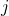.

Let’s see how this proposition works. Consider the adjacency matrix of the graph above: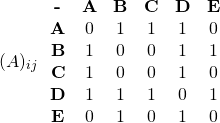With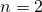we should find paths of length 2. So we first need to square the adjacency matrix: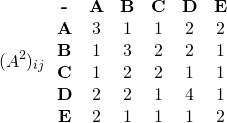Back to our original question: how to discover that there is only one path of length 2 between nodes A and B? Just look at the value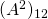, which is 1 as expected! Another example:, because there are 3 paths that link B with itself: B-A-B, B-D-B and B-E-B.

This will work with any pair of nodes, of course, as well as with any power to get paths of any length.

### Why does it work?

Now to the intuition on why this method works. Let’s focus onfor the sake of simplicity, and let’s look, again, at paths linking A to B., which is what we look at, comes from the dot product of the first row with the second column of:Now, the result is non-zero due to the fourth component, in which both vectors have a 1. Now, let us think what that 1 means in each of them:

•– first row -> first node (A) is linked to fourth node (D)
•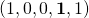– second column -> second node (B) is linked to fourth node (D)

So overall this means that A and B are both linked to the same intermediate node, they share a node in some sense. Thus we can go from A to B in two steps: going through their common node.

The same intuition will work for longer paths: when two dot products agree on some component, it means that those two nodes are both linked to another common node. For paths of length three, for example, instead of thinking in terms of two nodes, think in terms of paths of length 2 linked to other nodes: when there is a node in common between a 2-path and another node, it means there is a 3-path!

• Was this Helpful ?
• yes   no

## 2 thoughts on “Finding paths of length n in a graph”

1.gg says:

Does this algorithm really calculate the amount of paths?
By intuition i’d say it calculates the amount of WALKS, not PATHS ?

1.Stefano Ottolenghi says:

Uhm, why do you think vertices could be repeated?